## CNers have asked about a donation box for Cloudy Nights over the years, so here you go. Donation is not required by any means, so please enjoy your stay.# Finding the Optimal Sub-frame Exposure

Finding the Optimal Sub-frame Exposure Length for Astrophotography

Finding the Optimal Sub-frame Exposure Length for Astrophotography

Abstract

There has been a great deal of discussion about the optimal sub-frame exposure length and papers have been written on the subject. This paper discusses the issues of noise from all sources in astrophotography and attempts to find the optimal sub-frame exposure length from both the mathematics and empirical data. The current wisdom of simply overwhelming camera noise with light pollution noise as the optimal sub-frame exposure is found to be false. This paper shows that when shooting for the faintest details, target noise becomes the overwhelming problem and it cannot be reduced efficiently by stacking. The end result shows a formula to calculate the optimal sub-frame exposure based upon the camera’s noise, location’s light pollution noise, and total exposure. A number of interesting guidelines result from this formula.

Motivation

About once a month someone posts the question “What length should I use for my sub-frame exposures?” Conventional wisdom is that it depends upon the amount of light pollution and is based upon a formula from this paper by John C. Smith here: http://www.hiddenloft.com/notes/SubExposures.pdf. This seemed incorrect for at least three reasons.
1) Why would I care if the light pollution signal was 20 times the read noise? I am not imaging light pollution and will subtract it out of the final photo. I image targets and the target’s signal should overwhelm read noise.
2) What about quantization error? Cameras do not have infinite resolution and cannot report fractions of an ADU. They have whole number values and some faint target signals will only achieve 1 or 2 ADU if the exposure is too short.
2) My own empirical data did not support the formula. I got better low level detail when shooting longer than recommended sub-frames for the same total time.

Since my first read of Smith’s paper I have come to realize he was computing the noise in the light pollution signal is equal to the square root of the signal. So my first statement is wrong and he was trying to get light pollution noise to overwhelm the read noise. I am leaving 1) because my belief in it was part of the motivation for this research and does not affect the final results. The first part of this paper will cover in more detail what the paper above already covers but uses empirical data instead and also has a different goal.

Purpose

Stacking sub-frames is the preferred method for reducing noise over increasing exposure time because in addition to reducing camera and light pollution noise, it can also reduce other types of noise and non-random events that longer exposures cannot. Also shorter exposures are less prone to guide errors and if a sub-frame is bad, throwing it out has a smaller impact. However, we do not want to use exposures that start with a lower than necessary SNR. The goal is to find the optimal sub-frame exposure time defined as the point where the target’s SNR of stacking two sub-frames is within 5% the target’s SNR of exposing for twice as long. This is not the same as trying to find the point where camera noise adds only 5% to the total noise as the paper referenced above calculates and the difference will be shown later.

Basic Equations

Following are the basic equations used throughout the paper.

(1)Where: B = Bias of camera
DC = Dark Current of camera
EDC = Dark Current noise
R = Read Noise of camera
LP = Light pollution
ELP = Light pollution noise
Tgt = Target
ETgt = Target noise
t = exposure time
GainVign = Gain caused by vignetting (<= 1.0) of the optical tube or lens

(2)Equation 1 represents the equation for the value of the pixel after time t. All pixel values in this paper will be in ADU, Analog to Digital Units (i.e. the value read from the camera), and not in electrons. One thing missing from Equation 1 is the hot/cold pixel gain. The errors caused by hot/cold pixels are best handled through dithering and nearest neighbor type solutions. This paper deals with the 99+% pixels that do not have pixel gain significantly different from 1. Equation 2 represents the resulting noise error term when combining two independent noise error terms. Stacking two sub-frames is equivalent to combining two identical independent noise terms. This leads to
(2a)Stacking two sub-frames increases the target signal by 2 but noise only byso the resulting SNR is increased by, shown in Equation 2b.
(2b)Equation 2 also allows us to solve for E1 if you know E3 and E2.
(2c)All noise terms, expressed as Esubscript or R are expressed as the root mean square (RMS) of the error.

Now it is time to work on the noise. Since the CCD is a linear detector we can treat each term independently.

Camera Noise

Let’s first look at the camera’s contribution to signal and noise.

(3) Camera =Dark sub-frame subtraction will remove both the bias and the dark current signal so we are left with dark current noise and read noise. The formula for camera noise Ecamera is
(3a)We will not concern ourselves with the issues of dark current saturating the sensor and assume the camera’s manufacturer limits the maximum exposure to below this threshold. Both the read noise and dark current noise terms can be measured easily so we do not need the values from the manufacturer. Now what happens to our optimal exposure length if we only consider camera noise, ignoring light pollution? If we focus on just the read noise of the camera we get the. If we double the exposure to increase our signal by 2, the read noise remains constant so our SNR goes up by 2. We have already shown in Equation 2b that stacking two sub-frames only increases SNR by, therefore we should expose for as long as possible to increase SNR when considering only read noise. What about dark current noise? Since it increases at a rate of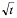, doubling the exposure or stacking two sub-frames are equivalent. So the camera’s optimal sub-frame exposure is as long as possible; or is it? A new question arises: Is there a point at which the dark current noise overwhelms the read noise such that the SNR of doubling the exposure is equivalent to the SNR of stacking two sub-frames? This is what we are trying to do with light pollution noise but let’s look at the camera in isolation.

Let’s define optimal for the camera the same way we defined optimal for light pollution. The optimal exposure for a camera is the point at which the SNR of combining two images is within 5% of the SNR of exposing twice as long.

Now at this point let me take a quick mathematical side trip. When combining two noise terms, how much larger does the first term have to be than the second term to reach the point where stacking and doubling are within 5%? Using Equations 2 and 2b we have
(4)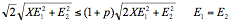where p is the percentage tolerance, 5%

The left hand side represents theincrease in noise by stacking and the right side represents the increase in noise by doubling the exposure. The error terms E1 and E2 are equal since we are trying to solve for the X multiplier. Since we know the right hand side will be the smaller term, it is the one that gets the (1 + p) term. Squaring both sides and just using E for the error term since they are all identical, we get

(5)Solving for X and skipping a bunch of in between steps we get

(6)So in Equation 2, thenoise term must be 4.38 times higher than theterm we are trying to overwhelm. What does this do to the resulting total error value E3? Plugging back into Equation 2 we get

(7)So we only need to expose until our measured total error to 2.32 times the error we are trying to overwhelm. Compare the above with the formula given by John C. Smith’s paper where he computed X=9.76. Using that value and solving for pwe get p=2.4%. So using a goal of read noise being 5% of the total noise reduces the error from stacking to just less than half of our stated goal. The choice of p is somewhat arbitrary but we will stick with 5%.

For my SBIG ST-10XE camera, I measured a read noise of 6.3 ADU RMS. Using the data from SBIG, they have a read noise of 8.8e RMS with a conversion of 1.3e/ADU, which gives a read noise of 6.8 ADU RMS. To measure the read noise I took 16 bias frames at CCD temperatures of -5C, -15C, and -25C. I then computed for each temperature the error (XPixel-X’Pixel) for the same pixel among the 16 frames. Finally I computed the RMS of all of the error terms over all of the frames and pixels, called Epixel. This is more accurate than taking one frame, averaging all pixels and computing Epixel from the overall average because of hot/cold pixel bias. The assumption is that a bias frame contains zero dark current noise, which seems reasonable. The results showed that read noise is independent of temperature.

Now I needed to find the dark current noise of the camera. The equation for total camera error is shown in Equation 3a. I took 1 minute, 5 minute, 10 minute and 20 minute dark frames at -15C, measured the total error from the frames and solved for dark current error using Equation 2c. The results were:
 CCD temperature = -15C 1 minute 5 minutes 10 minutes 20 minutes Ecamera measured 6.52 7.26 7.91 9.03computed from1.66 3.61 4.78 6.48 EDC/minute computed 1.66 1.64 1.51 1.45

The 5 minute shot goes up almost exactly by, and the 10 and 20 minute dark current noise values go up very close to, as predicted. The decreasing EDC term shows that dark current noise isn’t quite following theformula perfectly and that dark current gets slightly less noisy the longer the shot. After 20 minutes the dark current and read noise terms are effectively identical. From Equation 6 we know that we need to overwhelm the second term by 4.38 to achieve the optimal exposure so that means we should expose for 4.38*20 minutes = 87.6 minutes, longer than the 60 minutes maximum exposure of an SBIG camera. The pessimist view is my camera’s read noise is too high to get the optimal exposure below the maximum allowed exposure length. The optimist view is the dark current noise is sufficiently lower than read noise to not reduce maximum exposure. For another camera, its parameters would have to be measured and run through the equations.

Notice how the camera’s total noise increases as the exposure time increases. This will be important when solving for the optimal sub-frame exposure in the next section.

Light pollution Noise

Now we come to the heart of the paper. We have empirical values for camera noise and we have solved what it means to overwhelm a noise term with another noise term in Equations 6 and 7. We just need to measure the light pollution noise of the sky at a location. The light pollution signal adds uniformly to every pixel of the entire frame, ignoring gradients. The value of this signal is the average background value where no astronomical target exists (i.e. background of space) minus the camera bias. This value will simply be subtracted from every pixel when setting the black level so it is of no consequence. Smith’s paper uses an estimation of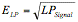, but I prefer to actually measure it to verify reality follows theory. The RMS of the actual error was measured across multiple frames from my research shots. If you want to measure it, I recommend taking one minute shots and use that as the unit of time T. It has the advantage of long enough to remove transient errors and most people shoot in units of 1 minute. You could use something shorter like 10, 20, or 30 seconds if you normally shoot in multiples of those lengths but the length must be long enough to overwhelm read noise.

At my Bortle orange level light polluted house, I took 45 1-minute shots, 27 2-minute shots and 9 5-minute shots of the same object, NGC7331, interleaved at 5 1-minutes, 3 2-minutes and 1 5-minute nine times. The RMS of the noise terms were
 CCD temperature= -15C 1 minute 2 minute 5 minute ETotal measured 16.5 23.5 31.5computed from15.2 22.6 30.7 ELP/minute 15.2 16 13.7 Avg. background pixel value measured across all frames, camera bias of 100 subtracted. ~245 ADU ~500 ADU ~1300 ADUin ADU 13.7 13.9 14.1

If we use the 1 minute ELP value as the basis, the 2 minute shots were slightly noisier and the 5 minute shots were less noisy but reasonably close. Estimating ELP usingis pretty close and more stable but might be a conservative and is theoretically the lowest possible value.

Note: When calculating the RMS of the error, it is critical that each sub-frame be normalized with each other using the average background value. The light pollution signal changes over time and if they are not normalized, the RMS goes sky high. For the 5-minute sub-frames I collected the average background ADU varied from 1200 to 1500. It also shows that a stacking program must also normalize the backgrounds before stacking to get the most SNR improvement.

Now given ELP and Ecamera, how long is the optimal sub-frame exposure? Using Equations 2 and 6 we get

(8)Solve for S, the sub-frame length
(9)The irony here is the camera’s dark current noise actually helps reduce the exposure time. Plugging in my local values where ELP is 15.2 from the table and EDC is the camera’s dark current noise = ~1.6 and R is the read noise which measured 6.3 we get

(9a)Equation 9 tells you how long to expose where stacking and lengthening the exposure are equivalent in reducing light pollution noise plus camera noise within 5% (i.e. overwhelmed read noise with light pollution noise). The units of S are whatever length of exposure you used to find ELP. So for the data above, using 2 minutes gives 41 seconds and 5 minutes gives 55 seconds. So there is a little disagreement on the exact length because there is variance in the variance when measuring the actual values.

Here is a table of minimum exposure lengths in minutes using Equation 9 for both ELP and ETotal based upon my camera’s dark current noise, EDC, and read noise, R, and may not be valid for other cameras.

 ELP/minute Min Exposure in minutes without dark current Double Read Noise = 12.6 ETotal/minute Min Exposure 18 0.53 0.54 2.13 18 0.61 17 0.60 0.60 2.38 17 0.70 16 0.67 0.68 2.69 16 0.80 15 0.76 0.77 3.06 15 0.94 14 0.88 0.89 3.50 14 1.11 13 1.01 1.03 4.05 13 1.34 12 1.19 1.21 4.74 12 1.67 11 1.41 1.44 5.63 11 2.14 10 1.70 1.74 6.78 10 2.88 9 2.08 2.15 8.32 9 4.21 8 2.61 2.72 10.45 8 7.15 7 3.37 3.55 13.49 7 18.67 6 4.51 4.83 18.03 5 6.31 6.95 25.23 4 9.37 10.87 37.47

Table SEQ Table * ARABIC 1 : Minimum Exposure in minutes where stacking and lengthening exposure are identical

ETotal is the photos’ total error (camera noise + light pollution noise) and will be used for the remainder of the paper because it is camera independent and it can be measured directly from the photos. As you can see, the minimum exposure remains quite short until the light pollution noise goes below the camera’s noise, at which point it takes a long exposure to dominate the camera’s larger noise term. Also the dark current signal is so small that it has almost no influence on the minimum exposure time. Look at what happens if we double the read noise of the camera. The exposure goes up by the square of the increase. Low read noise is very important if you want to stack as many frames as possible in a fixed amount of time. The ETotal stops at 7 because the total noise cannot be lower than the camera noise.

If you cannot measure the actual RMS of the light pollution error, then usingseems like a reasonable alternative. At worst case it calculates a value a bit lower than actual error and that is better than too high. I hope to create a user friendly version of the program I wrote that measures it from a bunch of FITS files. The current program requires changing the code to change the file names and is not the least bit user friendly. Some might take issue with estimating ELP from the entire photo since for the bright targets; ETarget is much higher than ELP. For the research shots I used this did not affect the measured value by enough to matter.

So I have now found the optimal exposure length for my house near zenith equals 45 seconds. Wait a minute! One of the reasons for doing this research was the fact that my empirical data did not match up with the predicted formula. I am now using an “improved” formula with the actual measured error values and it says to shoot even shorter? What is going on here?

Low Signal Error and Quantization

Hovering over the faintest parts of a galaxy arm in my combined 1 minute research shot, the value was barely 2 or 3 ADU above the background, which had an ETotal = ~2.5 ADU. In the combined 5 minute shot, the same pixels were easier to discriminate and about 15 ADU above the background with an ETotal = ~10.3. The signal strength of these faintest signals was about 3 ADU / minute. That is the issue.

In our zeal to overwhelm camera noise with light pollution noise, we forgot our true purpose: Find the length of time where the target’s SNR is within 5% when stacking or doubling exposure time. We left out the target’s noise term. The general assumption is ETgt is much smaller than ELP when TgtSignal << LPSignal so it has no impact but let’s look at it in more detail for all cases. The previous sentence in plainer English is “the general assumption is target noise is too small to matter when compared to light pollution noise when the target is much fainter than the light pollution”. From Equation 1, if we assume that all other noise terms are zero, then
(10) SNR =For objects with very strong signals like the Moon, planets, and high magnitude stars, the target noise overwhelms all other noise and the SNR is Equation 10 and the value is set by the transparency and seeing. Stacking is the only way to improve it and our exposure length is set by the target’s signal, not light pollution noise. For less strong signals there is a choice of shooting longer or stacking. At this level ETgt and ELP are on the same order of magnitude so we really only need the combination to overwhelm read noise, not an individual term. But for the weakest of signals, the ones the camera barely gets any ADU, target noise from quantization error and low probability becomes significant compared to target signal and light pollution noise is no longer the biggest concern. The entire concept of stacking / exposure doubling works equivalently at reducing noise because we assume that the signals have a normal distribution so the error is ~. That is only true if we collect enough signal data. A better model for weak signals is the Binomial distribution and Poisson distribution for small ?. I will not go into the gory details here and you can follow the links for a detailed explanation.

At only 1 ADU every 20 seconds, a 1 minute shot only has a ? = 3 (expected number of ADU / time frame), below normal distribution. To add to the error, a signal of 3.0 to 3.99 will also appear as a signal of 3 due to quantization error. To add insult to injury, signals with strength 1, 2, 4, and 5 ADU / minute are also below normal distribution and significant quantization error, which confuse the situation even more. The noise cannot simply be stacked away without a huge number of frames and quantization error still persists since we are at the level of a few ADU and the sensors do not report fractions of an ADU.

My 5 minute shot has a ?=15 (3 ADU / minute for 5 minutes) for the faint details. Right around ?=15, the Poisson distribution is more like a normal distribution and can be reasonably modeled that way. At ?=25 it is effectively normal and should be treated as such. So now we need yet another equation in the calculation of optimal sub-frame exposure, one that takes into account low signal error. We now have a new goal. We want to guarantee at least 15 ADU from the weakest signal, but how weak is that? If we double the exposure then we can get 15 ADU from a signal one half of the current weakest signal. Does that mean we need to expose as long as possible again? No, because we have a competing noise term from the camera and light pollution. At some point the noise is going to overwhelm 15 ADU. At a minimum we should expose to the point where the average background + ETotal equals the average background + minimum signal – ETotal, or where ETotal = ?/2. Since we are defining the minimum signal as ?=15 ADU, the ETotal should be 7.5. But can’t we reduce noise by stacking multiple sub-frames and so are stuck in an infinite loop? Time for us to do some more math heavy lifting.

No matter how many sub-frames you want to stack, there is going to be a limit in total exposure time, call it TTotal. The final combined stacked photo’s total noise term,will be
(11)Where: ETotal is the total noise for a shot of time T.
S is the sub-frame length in units of time T
Ttotal is the total time in units of time T

Equation 11 is a simplification and is valid if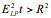(i.e. light pollution noise overwhelms camera noise in time t). We are no longer concerned with differentiating light pollution noise from camera noise and assume. Simplifying the Equation 11 gives

(12)To simplify the time units we will use unit of T = one minute making the whole equation in minutes. Playing around with different values of S we see that as we increase S, theterm goes up linearly so doubling S doubles. Since we also doubled the signal by doubling the exposure time, SNR remains constant. Only a change in TTotal will change the SNR. This is critical to understand. For all but the weakest signals, all combinations of sub-frame exposure and number of sub-frames to stack that add up to TTotal have equivalent SNR as long as the sub-frame exposure is greater than the minimum value where stacking and doubling the exposure are equivalent, given in Equation 9. Since we define equivalence by our choice of p, the exact definition of equivalence is a bit flexible.

Now we stated above that we wantto be less than ?/2, or 7.5. Using Equation 12 and putting ? back into the equation in case someone wants a different value for ? we get

(13)Solving for S we get
(14)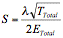Equation 14 is the equation for the optimal sub-frame exposure for TTotal that is as short as possible but leaves no reasonably detectable signal on the table. Plugging my location’s values into (14) for a 45 minute total exposure, we get

(15)This value is quite reasonable given my research shots. To verify Equation 14, let’s create a table of ETotal vs. total exposure to see what it recommends.
 ETotal/minute TTotal in minutes 60 120 180 240 300 360 18 3.23 4.56 5.59 6.45 7.22 7.91 17 3.42 4.83 5.92 6.83 7.64 8.37 16 3.63 5.13 6.29 7.26 8.12 8.89 15 3.87 5.48 6.71 7.75 8.66 9.49 14 4.15 5.87 7.19 8.30 9.28 10.16 13 4.47 6.32 7.74 8.94 9.99 10.95 12 4.84 6.85 8.39 9.68 10.83 11.86 11 5.28 7.47 9.15 10.56 11.81 12.94 10 5.81 8.22 10.06 11.62 12.99 14.23 9 6.45 9.13 11.18 12.91 14.43 15.81 8 7.26 10.27 12.58 14.52 16.24 17.79 7 8.30 11.74 14.37 16.60 18.56 20.33 6 9.68 13.69 16.77 19.36 21.65 23.72 5 11.62 16.43 20.12 23.24 25.98 28.46 4 14.52 20.54 25.16 29.05 32.48 35.58

Table SEQ Table * ARABIC 2 : Optimal sub-frame exposures in minutes

Here is a 3-d plot of the dataFigure SEQ Figure * ARABIC 1 : 3D plot of optimal exposures for RMS and Total Time

As you can see, it recommends between a 5 and 10 minute optimal sub-frame (60 minutes total in the front, 360 minutes total in the back) except in cases of shooting short total time in extreme light pollution or shooting very long total time in pristine skies with cameras that have exceptionally low camera noise. These times must also be checked against Table 1’s values for the minimum time. The results seem to match up with what most astrophotographers with SBIG cameras found empirically by trial and error; the optimal sub-frame length is generally between 5 and 10 minutes.

There is a practical limit to the lowest level signal that can be detected even if we ignored all light pollution and atmospheric extinction. My SBIG camera’s noise goes over 9 at 20 minutes and predicted as 15.6 at 60 minutes. At 1 hour exposures and reworking Equation 14 to solve for Ttotal, I only need 5 exposures to achieve all I could reasonably get out of the SNR, with a lowest level signal of 1 ADU / 4 minutes. A camera with even lower noise could theoretically go lower assuming we haven’t reached atmospheric extinction. There are also other practical considerations of tracking and seeing that might increase the minimum signal we can actually detect.

Equation 14 is a simplification based on the assumption that. The true equation for S is

(16)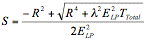Equation 16 can be used for all values of ELP and R and must be used when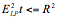.

Discussion

It seems pretty clear from the equations that it is a combination of the target’s attributes, read noise, and light pollution that decide on the appropriate exposure. Either the target’s exposure is limited by its strong signal or we are free to expose for a large amount of time but are limited at some point by light pollution noise. As was stated earlier, not all targets can actually take the optimal exposure. Obviously solar system objects, bright nebula, and clusters will need shorter shots to prevent blooming. Another consideration is the number of shots to be stacked. In a very dark site with very limited exposure time, the optimal exposure may be too long to get enough sub-frames to get a good distribution or deal with other types of noise. At that point you may be better off going with a shorter exposure to give enough sub-frames to stack and sacrificing some low level signal. However, choosing a shorter sub-frame exposure and still shooting the same or more total time gains nothing for stronger signals and loses the low level signal. Let me demonstrate this with an example.

Example 1

We are shooting at a location and camera combination that has an ETotal = 16. At this location we only need to shoot 48 seconds to be in the range where stacking and doubling exposure are equivalent. The target has a signal-strength of 150 ADU/minute. We first shoot 64 1-minute shots. Calculate the SNR of the target using a stacking method where we keep the target ADU value the same and reduce ETotal by the, like averaging.The SNR = 75 for the target. Let’s instead shoot 16 4-minute shots for the same total 64 minutes. What happens to the SNR? The signal goes up by a factor of 4 (4 times the exposure) and the noise goes up by the square root of 4.SNR remains constant for the target. We should expect this because by definition we are in the range where stacking and doubling exposure are equivalent with a sufficiently strong signal. We are free to choose any combination of exposure length and number of sub-frames that totals 64 minutes. If we are free to choose any combination, why not choose the one that discriminates the lowest possible signal? Equation 14 tells us the optimal combination among all combinations. This also leads to another interesting result. Let’s take Equation 14 and solve for TTotal as a function of fixed sub-frame length and noise.

(17)Table 3 shows the number of sub-frames to stack for a fixed sub-frame exposure and ETotal noise. The number of sub-frames has been rounded up to X+1 if over X+0.2 to make sure we get enough sub-frames.

 Sub-frame length in minutes ETotal/minute 1 2 3 4 5 6 7 8 9 10 18 6 12 18 23 29 35 41 46 52 58 17 5 11 16 21 26 31 36 41 47 52 16 5 9 14 19 23 28 32 37 41 46 15 4 8 12 16 20 24 28 32 36 40 14 4 7 11 14 18 21 25 28 32 35 13 3 6 9 12 15 18 21 24 27 30 12 3 5 8 11 13 16 18 21 23 26 11 2 5 7 9 11 13 15 18 20 22 10 2 4 6 7 9 11 13 15 16 18 9 2 3 5 6 8 9 10 12 13 15 8 1 3 4 5 6 7 8 9 11 12 7 1 2 3 4 5 6 6 7 8 9 6 1 2 2 3 4 4 5 5 6 7 5 1 1 2 2 3 3 3 4 4 5 4 1 1 1 1 2 2 2 3 3 3

Table SEQ Table * ARABIC 3 : Optimal number of sub-frames to stack

As you can see from the values, as noise increases, so does the number of sub-frames that must be stacked to achieve the practical minimum signal. The optimal number is also effectively the maximum number of sub-frames. Shooting more than the optimal number of sub-frames will improve SNR over camera and light pollution noise but for no reason, called over-stacking or the law of diminishing returns. By definition we have already achieved the practical minimum signal that can be distinguish from the background and lower level signals are lost in their own noise no matter how many additional sub-frames are stacked. Shooting additional sub-frames is equivalent to increasing TTotal. The new TTotal has a different (longer) optimal sub-frame exposure and we could have achieved the same SNR increase and picked up fainter signals by using it as shown in Example 1. The only reasons to shoot more sub-frames than recommended are cosmic rays, dithering requires it to fix hot/cold pixels, or to have a few extra sub-frames in case of tracking errors, satellites, airplanes or periods of bad seeing with the idea that some of them will have to be thrown out.

What about shooting exposures that are longer than the optimal, equivalent to increasing the value of ? in Equation 14? What do we lose if the optimal exposure is 4 minutes for 16 sub-frames and ETotal=15, but we decide instead to expose those 16 sub-frames for 8 minutes? The noise in the combined sub-frames will have gone from 7.5 tobecause we doubled the exposure and are still stacking the same number of sub-frames. The weakest signal we can clearly discriminate is twice the noise value so it went from 15 ADU toADU in 8 minutes or 2.65 ADU/minute. Now let us look at the optimal exposure for TTotal = 128 minutes (16 sub-frames * 8 minutes) and ETotal=15. Using Equation 14 we get S = 5.7 minutes. We have already shown that the SNR for stronger signals is only dependent on TTotal so the SNR is the same. What about the weakest signal we can discriminate? The optimal value has 15 ADU / 5.7 minutes = 2.65 ADU / minute so the lowest signal remains identical. The result of shooting longer than optimal exposures for the same TTotal is effectively identical across the entire signal spectrum and there is no advantage to it. The true effect is moving the weakest signal closer to normal distribution, but if you already decided ?=15 or 10 or 20 is close enough to normal distribution then why make it more normal? What we lose by going longer than optimal is the larger number of sub-frames that achieves the equivalent result. The optimal exposure has 22.5 (round up to 23) sub-frames to stack instead of just 16. More sub-frames mean a bigger reduction in other types of noise such as cosmic rays and hot/cold pixels and we still achieve the same signal quality. Why shoot 16 sub-frames when we can shoot 23 in the same amount of time with equivalent results?

We can also reverse the question in the previous paragraph for 8 minute exposures to “What do we lose if we only image 16 sub-frames instead of the optimal 32?” By stopping imaging early we did not achieve the lowest level signal possible for the 8 minute exposure. To achieve the identical low-level signal we could have reduced the exposure to 5.7 minutes and imaged 23 sub-frames instead of 16. Not imaging the full optimal number of frames means there was a shorter exposure that would have generated equivalent results.

At this point you may wondering what exactly is the difference between the light pollution limited exposure given in Equation 9 and the optimal sub-frame exposure based on the target and light pollution combination in Equation 14 so let’s take a detailed look. Equation 9 sets the exposure length and then reduces noise by the square root of the number of frames stacked. If you want to double the SNR, stack 4 times as many frames. Low-level signals will be seen after the noise is driven below them. Eventually the noise will be driven down to 2 RMS or even <1 RMS. Equation 14 takes a completely different approach. It assumes that signals below ?(=15) ADU will not be easily discriminated and computes the exposure length and number of frames to stack for TTotal so the final photo will have exactly ?/2 RMS error, no more and no less. If you want to double the SNR, you must shoot twice as long (doubles signal and increases noise by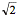) for twice as many frames (decreases noise by). The problem with the first approach is that low-level signals do not increase their SNR bybut something less than that. Also the law of diminishing returns kicks in when driving the noise error so low. Once the error term gets down to around 2, quantization error reduces the effectiveness of stacking. By keeping the final noise at ?/2, the optimal sub-frame exposure is always in the range where noise is reduced by the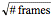.

Examine how the two approaches make you think about the problem of noise. Limiting exposure because of light pollution makes you think that 1) you can simply stack your way to any level of detail or 2) light pollution greatly limits the detail. Both are false. The optimal exposure makes you think in terms of length of exposure required to obtain the desired level of detail in the target and that light pollution determines how many frames to stack to drive the noise error down to ?/2. Light pollution becomes the enemy of total time and could even prevent the capture due to light pollution saturation. Look at Table 3 for a 10 minute exposure. A location that has strong light pollution of ETotal=17 requires about 3 times as many frames to stack to drive the noise to ?/2 and generate the same end result as it does at a dark site where ETotal=10. Over 8.5 hours versus 3 hours because 10 minute exposures at ETotal=17 have a noise of ~54 RMS and at ETotal=10 they have a noise of ~31.8 RMS. Image details are not limited by noise; they are limited by the length of exposure. You can always stack whatever number of frames necessary to drive the error to ?/2 but stacking a vast number of frames will not allow you to see signals you never sufficiently captured.

How does the optimal exposure length compare with the minimum exposure length? Let’s take another very detailed look at Equation 9. Table 4 shows the minimum exposure in minutes for 3 different read noise values, 4, 7, and 15 in various ELP locations and the resulting ETotal.

 Read Noise ELP/minute 18 17 16 15 14 13 12 11 10 9 8 7 4 0.22 0.24 0.27 0.31 0.36 0.41 0.49 0.58 0.70 0.87 1.10 1.43 ETotal 18.44 17.46 16.49 15.52 14.56 13.60 12.65 11.70 10.77 9.85 8.94 8.06 7 0.63 0.70 0.79 0.89 1.01 1.16 1.34 1.57 1.85 2.21 2.68 3.30 ETotal 19.31 18.38 17.46 16.55 15.65 14.76 13.89 13.04 12.21 11.40 10.63 9.90 15 2.64 2.92 3.23 3.60 4.02 4.52 5.11 5.80 6.61 7.58 8.72 10.06 ETotal 23.43 22.67 21.93 21.21 20.52 19.85 19.21 18.60 18.03 17.49 17.00 16.55

Table SEQ Table * ARABIC 4: Minimum Exposure for Read Noise

As you can see, the minimum exposure time goes up by the square of the read noise. What does it mean if we cannot to expose for the minimum time? All the minimum exposure indicates is the exposure length where light pollution noise overwhelms camera read noise such that stacking and doubling the exposure are equivalent. Exposing below that minimum simply means we would get better SNR improvement by exposing longer as opposed to stacking so it will taking longer total time, i.e. not 100% efficient with imaging time. Also high read noise cameras do not benefit nearly as much from a dark site’s lower light pollution noise. The optimal exposure equation does not care about the minimum and computes the breakdown of exposure and sub-frames for TTotal that creates the final photo with ?/2 error RMS.

Looking at Table 4, for a read noise of 15 and ELP=13, ETotal is 20 and the minimum exposure is 5.8 minutes. If you only want to image for 60 minutes, the optimal exposure is 3.9 minutes using Equation 16 because, which is below the minimum. At this point we are better off trying to image at the minimum exposure than the optimal because the SNR of the final image will be higher. However, the minimum is set by our choice of p=5%. What happens is we are more flexible and set it to p=10%, the equivalent of allowing read noise to be almost 24% of the total noise? The minimum exposure drops to 2.5 minutes, below our optimal value. Using the 3 exposure lengths of 2.5 minutes, 3.9 minutes, and 5.8 minutes, the minimum signal and SNR for TTotal =60 minutes are shown in Table 5.
 Exposure Min Signal ADU/minute SNR for 10 ADU/minute 2.5 6 4.8 3.9 3.85 5.1 5.8 3.7 5.4

Table SEQ Table * ARABIC 5: Min signal and SNR for 60 minute total exposure

It looks like p=10% might not be such a bad choice for the definition of “equivalence”. We lose an additional 10% off the SNR and the minimum signal does almost double but the minimum exposure is less than half. We cannot extend this logic much farther because the curve takes a sharp dive and we will lose significant SNR and the minimum signal keeps going up if we expose much shorter. Besides the optimal exposure is normally much higher than the minimum exposure for any reasonable TTotal except when the camera has extremely high read noise at a very dark site. For this case the results of the optimal exposure are very close to the results of the minimum exposure when p=5% but with 15 sub-frames instead of only 10.

The optimal sub-frame calculation still works even if we are well below the minimum exposure in the form of Equation 18 that computes the number of sub-frames to stack for a given exposure length and ETotal. Regardless of why ETotal = 22, even 100% read noise and 0% light pollution noise, the number of frames to stack tells you when you have achieved the lowest signal possible from the exposure length by driving the noise down to ?/2. Stacking more frames will not get any lower target signals and shortly will no longer increase SNR at a rate of.

“How do these equations help us image better?”

There are several ways to look at the results of these equations. The main use is to answer the question: “How long should my sub-frame exposures be if I only want to have a total exposure of 120 minutes?” Table 2 will give the answer. This answer is the optimal breakdown between longer exposures and more sub-frames for a fixed time assuming it is longer than the minimum exposure. Shorter exposures will result in a lower quality image for low level signals with no improvement in SNR for the stronger signals and longer exposures will be effectively identical but with fewer sub-frames to stack.

Another way is to fix the exposure length, either because that is all the target needs or that is all it can take before blooming, and compare how many sub-frames are necessary at different locations to achieve equal quality. This can be useful for astrophotographers whose home is light polluted but their dark site requires significant travel. Table 3 can be used to determine if the extra travel time could be used to collect more sub-frames at home and generate the equivalent result. In my own case the travel time one way is about 60 minutes plus 20 more minutes to tear down. Ignoring travel time to the location and setup, which are in the light, I could gain another 80 minutes of exposure and be much warmer by staying home. The ETotal at my home is ~16 and the “dark” site, a border Bortle yellow/green, is ~12. Using Table 3 for a 5 minute exposure, I would need to shoot an extra 50 minutes per object at home. During the week it definitely pays to stay home but it might be worth the travel time on the weekend if I plan to image for 4+ hours.

One final way to use the equations is to fix the number of sub-frames to stack. There are papers on the subject of how many sub-frames are necessary to deal with other forms of noise. If stacking 16 sub-frames gives the best overall reduction in noise not associated with the camera and light pollution, what is the correct sub-frame exposure length? Reworking Equation 17 gives

(18)where F is the number of sub-frames to stack.

 Total sub-frames to stack ETotal/minute 5 8 12 16 20 24 32 18 0.87 1.39 2.08 2.78 3.47 4.17 5.56 17 0.97 1.56 2.34 3.11 3.89 4.67 6.23 16 1.10 1.76 2.64 3.52 4.39 5.27 7.03 15 1.25 2.00 3.00 4.00 5.00 6.00 8.00 14 1.43 2.30 3.44 4.59 5.74 6.89 9.18 13 1.66 2.66 3.99 5.33 6.66 7.99 10.65 12 1.95 3.13 4.69 6.25 7.81 9.38 12.50 11 2.32 3.72 5.58 7.44 9.30 11.16 14.88 10 2.81 4.50 6.75 9.00 11.25 13.50 18.00 9 3.47 5.56 8.33 11.11 13.89 16.67 22.22 8 4.39 7.03 10.55 14.06 17.58 21.09 28.13 7 5.74 9.18 13.78 18.37 22.96 27.55 36.73 6 7.81 12.50 18.75 25.00 31.25 37.50 50.00 5 11.25 18.00 27.00 36.00 45.00 54.00 72.00 4 17.58 28.13 42.19 56.25 70.31 84.38 112.50

Table 6: Optimal sub-frame exposure in minutes for fixed number of sub-frames

This is a very powerful use of the equation because there are many reasons to use a specific number of sub-frames, or at least X sub-frames. Table 6 gives the optimal exposure for the camera and location’s light pollution noise to get the maximum quality out of those sub-frames. Shooting shorter exposures does not achieve the minimum signal possible (over-stacking). Shooting longer, while creating an identical photographic result, does not meet the stated goal of the shortest possible exposure to maximize the number of sub-frames. For my house at ETotal = 16 and shooting 16 sub-frames, I should use a sub-frame exposure of ~4 minutes. At my dark site with ETotal = 12, I should shoot ~6 minute sub-frames. Notice that the exposure time goes up linearly with the number of sub-frames to stack. If you double the number of sub-frames to stack, you should also double the exposure time, which increases total exposure time by a factor of 4.

The minimum exposure equation is necessary to determine if the optimal exposure is valid but how else could we use minimum exposure equation? Let’s look at a situation where I have a target, like a bright nebula, where I can only expose for 1 minute before blooming is an issue. From Table 1, I can get as low as ELP = 13 (or ETotal = 15) for my camera before I am below even the minimum exposure. What happens if I go to a really dark site? Noise keeps going down but I am no longer “optimal” because I could have achieved better SNR by increasing the length of the exposure instead of just stacking more sub-frames. However I have already stated I cannot shoot longer so that is not an option and am now in an under-exposure situation. The target is simply too bright to make optimal use of the dark site’s lower light pollution noise because of the read noise of the camera. This leads to another interesting guideline. If your dark site requires travel, choose targets that can take at least as much exposure as necessary to overwhelm camera noise. The minimum exposure becomes a guideline to the appropriate location for the exposure, not the length. Don’t waste your precious dark site minutes on targets that you can shoot at home with equivalent results. This is obvious when talking about solar system objects and bright stars but it also applies to DSOs. Now the corollary to this is “Results will always be at least as good at a darker site because of the lower light pollution noise”. There is no object that would be better shot in light pollution than a dark site regardless of exposure length chosen, but they might be effectively equal. DSOs also need less total exposure at a darker site to achieve the same SNR as the light polluted site so time is another factor in favor of the dark site.

With all of the equations and discussion so far, we should be able to answer the question “What is the actual impact of light pollution on astrophotography?” Light pollution has the following two effects.
1. Limits the maximum exposure before light pollution saturates the sensor.
2. Increases the noise in each sub-frame.

The first effect limits the lowest level signal obtainable in a light polluted location compared to a darker location because going “deeper” requires longer exposures. The second effect increases the number of sub-frames to stack to obtain the equivalent SNR of a darker site. Some view the second effect as reducing required exposure time and apply the converse to a dark site, which increases the required exposure time. Exposure length can be anything to the limits of saturation. There is no required minimum or maximum exposure time but there is a suggested minimum below which the SNR is lower than nominal. I hope you will see that exposure time is set by the level of detail you want from the target and not by some arbitrary ratio of light pollution to read noise. Strong light pollution simply means you will have to put a larger amount of total time into the object to get the same SNR and may not be worth the effort.

There may be some disagreement as to the exact value of ? to be used, which will affect the sub-frame values. Poisson distribution starts becoming normal at ? = 5 and a case can be made for using an aggressive value of ? = 10 (shoot shorter, stack more) or a very conservative ? = 25 (shoot longer, stack less). This paper uses p = 5% as a measure of equivalence but something closer to 10% might be a better choice. There might also be some reasonable disagreement about the necessity of shooting at the optimal combination given time constraints and other types of noise. Plenty of targets have sufficient dynamic range that it isn’t always worth going after the absolute maximum detail available at the edges. Equation 14 simply states how to achieve the theoretical maximum for a given camera, location’s light pollution, and how much time is to be put into the target but we are talking about fractions of a difference. Exposing a little longer, a little shorter, or stacking a few extra frames will not result in much change in the final photo. The key is that stopping at the minimum exposure when the optimal is twice that value makes a big difference. Use equations 9 and 14 to help make the most efficient use of your time under the stars. Table 6 is very useful if you like to shoot a specific number of sub-frames and do not want to shoot below the dark site’s potential. Table 3 shows when image quality is being lost from over-stacking. In the end there is wiggle room at the margins but the overall guidelines remain intact.

Conclusion

Our initial goal of simply overwhelming camera noise with light pollution noise did not produce the optimal sub-frame exposure for faint objects. It incorrectly ignored target noise and signal probability in low level signals where it became the dominant problem and cannot be solved by stacking more sub-frames. Also we may need to relax the definition of “overwhelm” for a camera with high read noise. Equation 9 is a recommendation for the minimum exposure; below which imaging is not as efficient at noise reduction. It is a useful guideline to determine the most effective location at which a target should be imaged if imaging at multiple sites and time is a premium.

The equation to find the optimal sub-frame exposure for capturing faint objects is defined aswhere ?=15. This gives the optimal combination of exposure length and number of sub-frames for total time TTotal that result in the lowest possible detectable target signal levels. Increasing the total exposure will allow even fainter target signals to be detected but there are practical limits. The equation can be changed around to fix either TTotal, sub-frame exposure, or number of sub-frames and solve for the optimal value of the other two. Use Tables 2, 3, and 5 as guidelines to get the best combination of sub-frame exposure, total time, and number of sub-frames to stack for a given target at a specific location. If you prefer to give up a little SNR and minimum signal to get more sub-frames then use p=10% and ?=10.

Using the minimum exposure and optimal exposure equations wisely can increase the efficiency of your time under the stars.

Further Research

A useful research project is to find out how small the atmospheric noise can get when shooting from some of the best places on Earth. It would be interesting to find out that as amateurs (using that term very loosely considering the incredible quality that is achieved) really start spending the money and effort to set up remote observatories in exceptional locations if camera noise becomes the dominant problem and we need a breakthrough in low-noise cameras for the next level of quality.

Another suggestion is a way to measure atmospheric extinction such that a formula can predict using camera A on telescope B at location C, the maximum useful exposure time is X minutes. This is a rather involved project because it has to take into account the actual telescope parameters and its effect on low-level signal detection. This would be of significant value for setting expectations and preventing astrophotographers from wasting hours trying to achieve the impossible. It would also point out the weakest link in the chain that gives the most improvement when upgrading.

• Bob R., PeterWar, Iksobarg and 6 others like this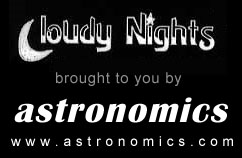Cloudy Nights LLC Cloudy Nights Sponsor: Astronomics